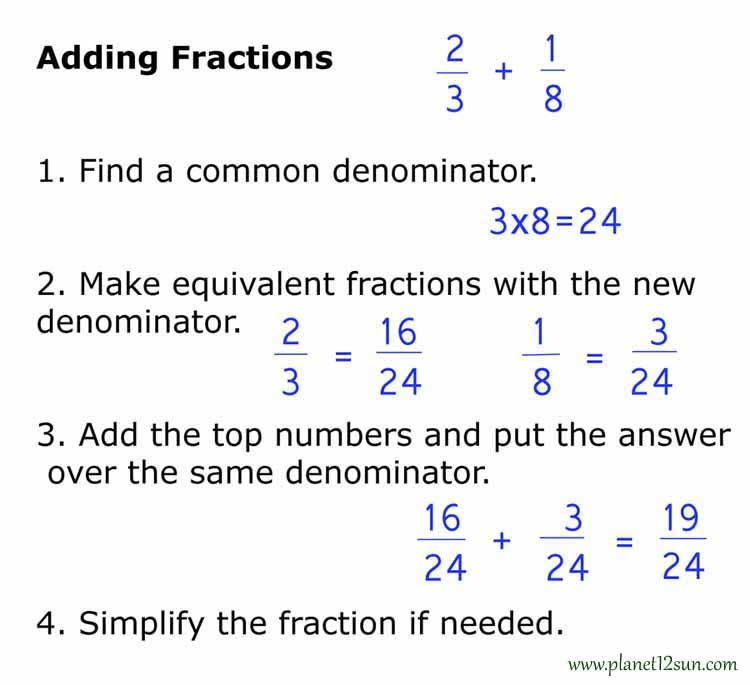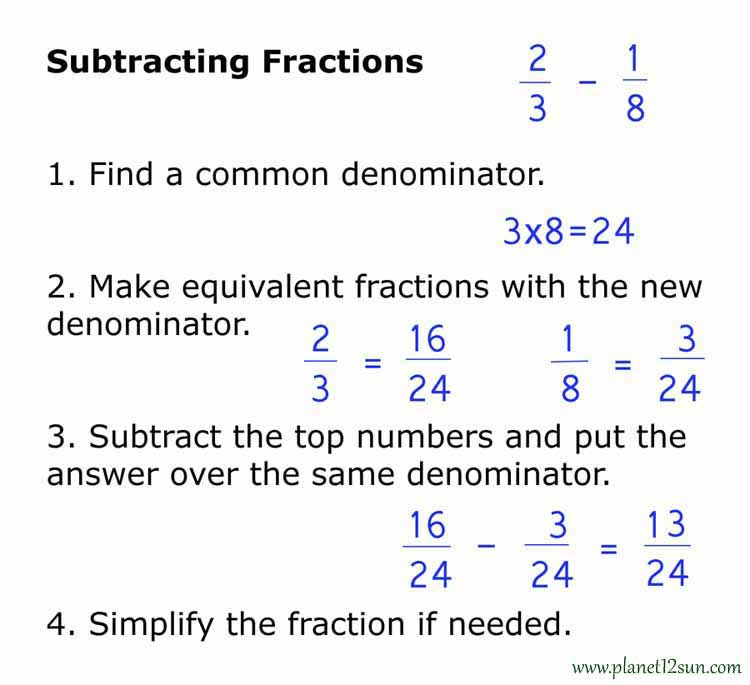## Fractions

### What is a fraction?

A fraction represents part of a whole. When something is broken up into a number of parts, the fraction shows how many of those parts you have.

Look at the pictures to see how the whole of a circle, rectangle and even liquid can be broken up into different fractions.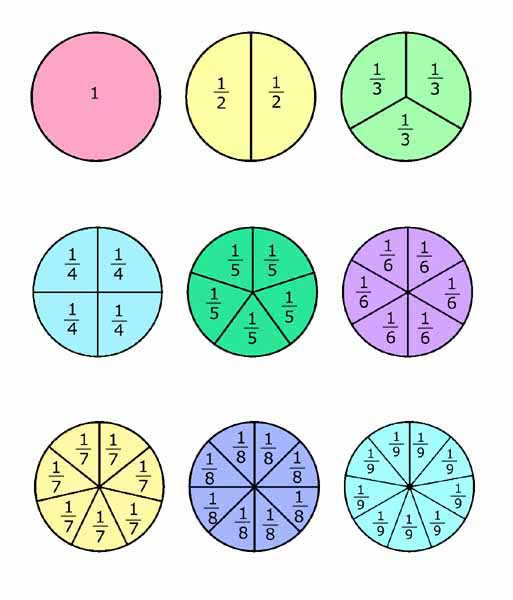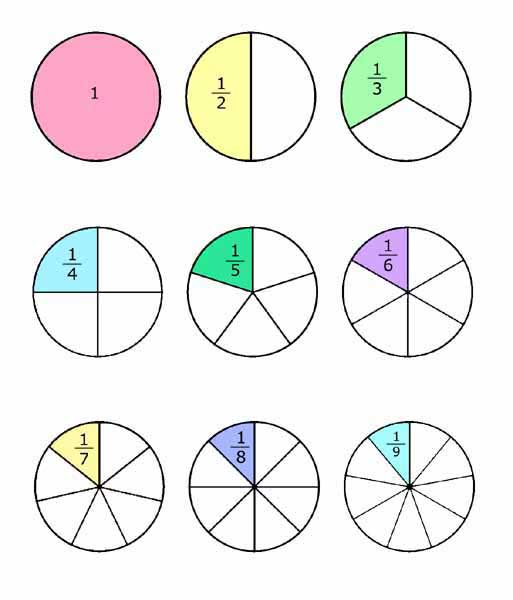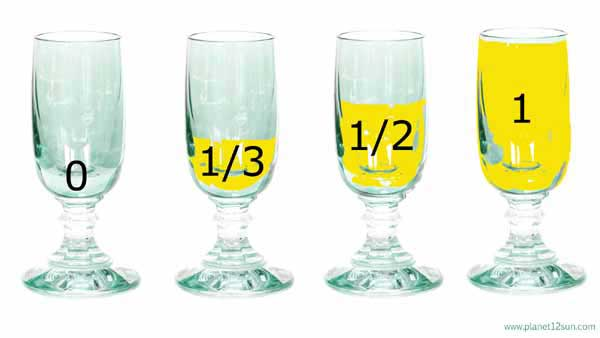### Numerator and Denominator

When writing a fraction there are two main parts: the numerator and the denominator. The numerator is how many parts you have. The denominator is how many parts the whole was divided into. Fractions are written with the numerator over the denominator and a line in between them.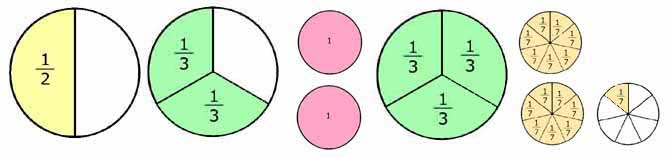### How to Read Fractions or Write them in Words

Write the numerator of a fraction spelled out in words exactly as it appears as a numeral. With the fraction 3/5, write the numerator as "three."

When reading fractions it is customary in English to pronounce the denominator using the corresponding ordinal number in plural if the numerator is not one, as in "fifths" for fractions with a 5 in the denominator. Thus, 3/5 is rendered as three fifths.

The exception to this is when the denominator equals two. For example, consider the fraction 1/2. You would never write this as "one- second." Instead, you would write "one-half."

You can write a denominator of "four" in two different ways: as fourths or as quarters. For example, you could express 3/4 correctly as either "three-fourths" or as "three-quarters."### Equivalent Fractions

Sometimes fractions may look different and have different numbers, but they are equivalent or have the same value.
One of the simplest examples of equivalent fractions is the number 1. If the numerator and the denominator are the same, then the fraction has the same equivalent value as 1.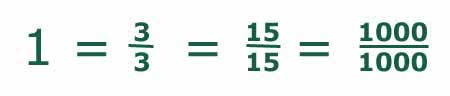Here are some equivalent fractions for 2/3. The equivalent fractions are all multiples of 2/3. Take 4/6 for example. 2x2 = 4 and 2x3 = 6.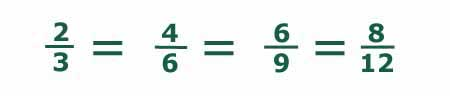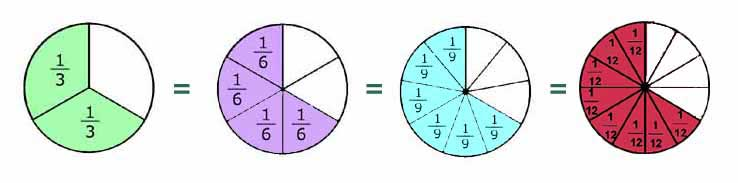### Types of Fractions

1. Proper Fractions - A proper fraction is one where the numerator is less than the denominator. A proper fraction is always less than one.
examples: 1/2, 3/5, 15/100...

2. Improper Fractions - An improper fraction is one where the numerator is greater than the denominator. An improper fraction is always greater than one.
examples: 4/2, 5/3, 7/1...

3. Mixed Fractions - A mixed fraction has both a whole number part and a fractional part.
examples: 2 1/7, 7 1/2, 1 2/3...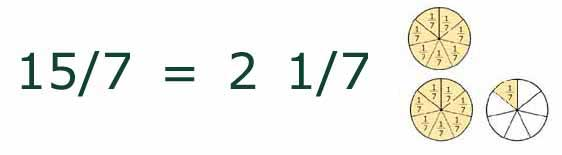To convert an improper fraction to a mixed fraction, follow these steps:

Divide the numerator by the denominator.
Write down the whole number answer.
Then write down any remainder above the denominator.

example: Convert 11/4 to a mixed fraction.
11 ÷ 4 = 2 with a remainder of 3.
Write down the 2 and then write down the remainder (3) above the denominator (4).
11/4 = 2 3/4

If there is no remainder, then the result is a whole number.

You can turn whole numbers into fractions by putting them over 1. (2 = 2/1, 7=7/1, 100=100/1...)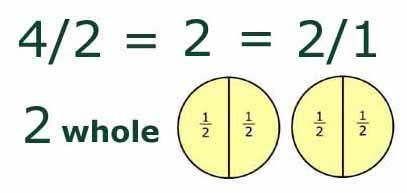### Decimals

When decimal points are used in numbers, the number to the right of the decimal point is a type of fraction. Depending on the place value it can be 1/10, 1/100, 1/1000 or some other factor of 10.
examples:
0.4 = 4/10
0.15 = 15/100

### Percents

Another type of fraction is the percent. The "percent" is a fraction with a denominator of 100. When you say 50% it is the same as saying 50/100.
examples:
10% = 10/100
25% = 25/100

### Simplifying Fractions

Simplifying (or reducing) fractions means to make the fraction as simple as possible. Try to evenly divide (only whole number answers) both the top and bottom of the fraction by GCF, or any number, for example 10, 9, 8, 7, 6 ... etc, until you can't go any further.

(The Greatest Common Factor GCF of some numbers, is the largest positive integer that divides the numbers without a remainder. Like, the GCF of 10,15, and 25 is 5.)

example 1: simplify the fraction 16/24
Divide both, the top and bottom by 2 until you can't go any further.
16/24 = 8/12 = 4/6 = 2/3
Or divide both, the top and bottom by 8.
16/24 = 2/3
Or divide both the top and bottom by 4.
16/24 = 4/6, then divide both the top and bottom by 2.
4/6 = 2/3

example 2: simplify the fraction 90/360
Divide both, the top and bottom by 10.
90/360 = 9/36
Divide both, the top and bottom by 9.
9/36 = 1/4

### Multiplying Fractions

1. Multiply the top numbers (the numerators).
2. Multiply the bottom numbers (the denominators).
3. Simplify the fraction if needed.

example:
2/3 x 5/6 = 10/18 = 5/9

### Dividing Fractions

1. Turn the second fraction (the one you want to divide by) upside down (this is now a reciprocal).
2. Multiply the first fraction by that reciprocal.
3. Simplify the fraction if needed.

example:
1/2 ÷ 1/6 = 6/2 = 3/1 = 3

1. Find a common denominator. (The bottom numbers have to be the same.)
2. Make equivalent fractions with the new denominator.
3. Add the top numbers (numerators) and put the answer over the same denominator.
4. Simplify the fraction if needed.

### Subtracting Fractions

1. Find a common denominator. (The bottom numbers have to be the same.)
2. Make equivalent fractions with the new denominator.
3. Subtract the top numbers (numerators) and put the answer over the same denominator.
4. Simplify the fraction if needed.